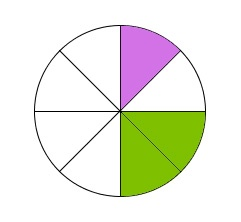# Students 6630

There are 26 pupils in the 7th grade, of which 10 are girls. Write down the ratio of the number of girls to the number of students. Adjust the ratio of this task so that the numbers are as small as possible.

p =  5:13

### Step-by-step explanation:Did you find an error or inaccuracy? Feel free to write us. Thank you!

Tips for related online calculators
Need help calculating sum, simplifying, or multiplying fractions? Try our fraction calculator.
Check out our ratio calculator.